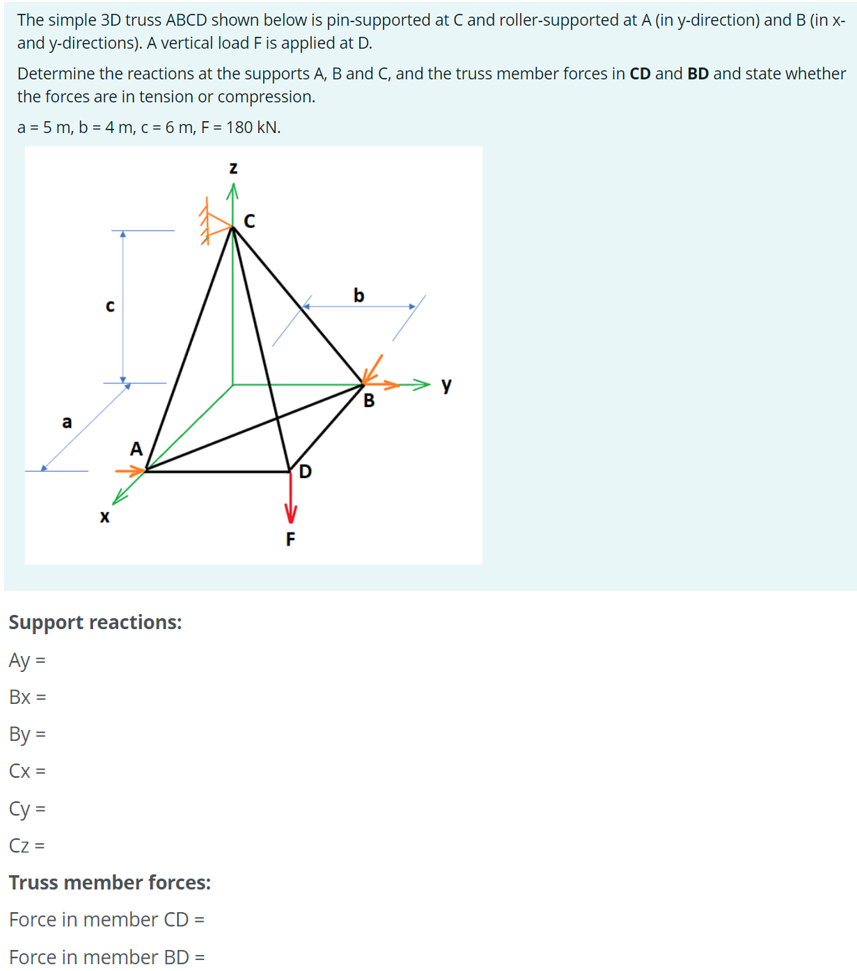# Question The simple 3D truss ABCD shown below is pin-supported at C and roller-supported at A (in y-direction) and B (in x- and y-directions). A vertical load Fis applied at D. Determine the reactions at the supports A, B and C, and the truss member forces in CD and BD and state whether the forces are in tension or compression. a = 5 m, b = 4 m, c = 6 m, F = 180 kN. Z b с y B а A F Support reactions: Ay = Bx = By = Cx= Cy = Cz = Truss member forces: Force in member CD = Force in member BD =